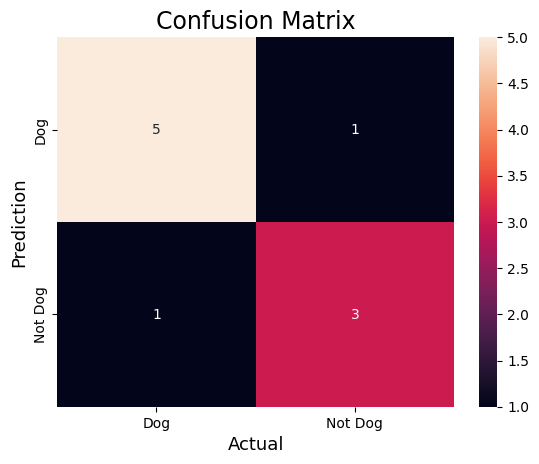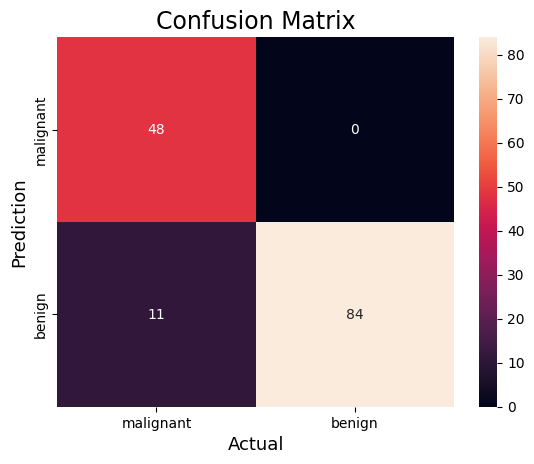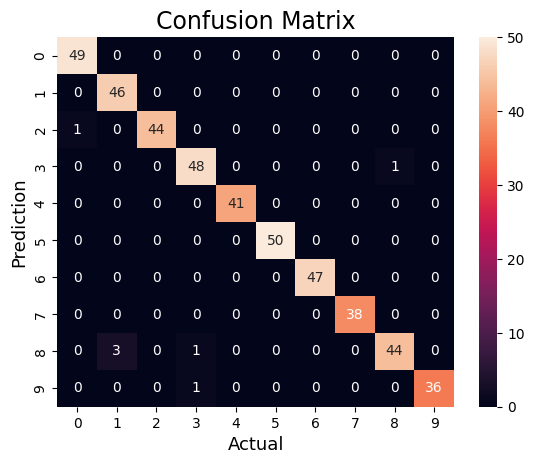GeeksforGeeks App
Open AppBrowser
Continue

# Confusion Matrix in Machine Learning

In machine Learning, Classification is the process of categorizing a given set of data into different categories. In Machine Learning, To measure the performance of the classification model we use the confusion matrix.

## Confusion Matrix

A confusion matrix is a matrix that summarizes the performance of a machine learning model on a set of test data. It is often used to measure the performance of classification models, which aim to predict a categorical label for each input instance. The matrix displays the number of true positives (TP), true negatives (TN), false positives (FP), and false negatives (FN) produced by the model on the test data.

For binary classification, the matrix will be of a 2X2 table, For multi-class classification, the matrix shape will be equal to the number of classes i.e for n classes it will be nXn.

A 2X2 Confusion matrix is shown below for the image recognization having a Dog image or Not Dog image.

• True Positive (TP): It is the total counts having both predicted and actual values are Dog.
• True Negative (TN): It is the total counts having both predicted and actual values are Not Dog.
• False Positive (FP): It is the total counts having prediction is Dog while actually Not Dog.
• False Negative (FN): It is the total counts having prediction is Not Dog while actually, it is Dog.

### Example

• Actual Dog Counts = 6
• Actual Not Dog Counts = 4
• True Positive Counts = 5
• False Positive Counts = 1
• True Negative Counts = 3
• False Negative Counts = 1

Confusion Matrix

### Implementations of Confusion Matrix in Python

Steps:

• Import the necessary libraries like Numpy, confusion_matrix from sklearn.metrics, seaborn, and matplotlib.
• Create the NumPy array for actual and predicted labels.
• compute the confusion matrix.
• Plot the confusion matrix with the help of the seaborn heatmap.

## Python3

 #Import the necessary librariesimport numpy as npfrom sklearn.metrics import confusion_matriximport seaborn as snsimport matplotlib.pyplot as plt #Create the NumPy array for actual and predicted labels.actual    = np.array(  ['Dog','Dog','Dog','Not Dog','Dog','Not Dog','Dog','Dog','Not Dog','Not Dog'])predicted = np.array(  ['Dog','Not Dog','Dog','Not Dog','Dog','Dog','Dog','Dog','Not Dog','Not Dog']) #compute the confusion matrix.cm = confusion_matrix(actual,predicted) #Plot the confusion matrix.sns.heatmap(cm,            annot=True,            fmt='g',            xticklabels=['Dog','Not Dog'],            yticklabels=['Dog','Not Dog'])plt.ylabel('Prediction',fontsize=13)plt.xlabel('Actual',fontsize=13)plt.title('Confusion Matrix',fontsize=17)plt.show()

Output:Confusion Matrix

#### From the confusion matrix, we can find the following metrics

Accuracy:  Accuracy is used to measure the performance of the model. It is the ratio of Total correct instances to the total instances.For the above case:

Accuracy = (5+3)/(5+3+1+1) = 8/10 = 0.8

Precision: Precision is a measure of how accurate a model’s positive predictions are. It is defined as the ratio of true positive predictions to the total number of positive predictions made by the modelFor the above case:

Precision = 5/(5+1) =5/6 = 0.8333

Recall: Recall measures the effectiveness of a classification model in identifying all relevant instances from a dataset. It is the ratio of the number of true positive (TP) instances to the sum of true positive and false negative (FN) instances.For the above case:

Recall = 5/(5+1) =5/6 = 0.8333

F1-Score: F1-score is used to evaluate the overall performance of a classification model. It is the harmonic mean of precision and recall,For the above case:

F1-Score: = (2* 0.8333* 0.8333)/( 0.8333+ 0.8333)  = 0.8333

## Python3

 #Import the necessary librariesfrom sklearn.datasets import load_breast_cancerfrom sklearn.model_selection import train_test_splitfrom sklearn.tree import DecisionTreeClassifierfrom sklearn.metrics import confusion_matriximport seaborn as snsimport matplotlib.pyplot as pltfrom sklearn.metrics import accuracy_score, precision_score, recall_score, f1_score # Load the breast cancer datasetX, y= load_breast_cancer(return_X_y=True)X_train, X_test, y_train, y_test = train_test_split(X, y,test_size=0.25) # Train the modeltree = DecisionTreeClassifier(random_state=23)tree.fit(X_train, y_train) # preductiony_pred = tree.predict(X_test) # compute the confusion matrixcm = confusion_matrix(y_test,y_pred) #Plot the confusion matrix.sns.heatmap(cm,            annot=True,            fmt='g',            xticklabels=['malignant', 'benign'],            yticklabels=['malignant', 'benign'])plt.ylabel('Prediction',fontsize=13)plt.xlabel('Actual',fontsize=13)plt.title('Confusion Matrix',fontsize=17)plt.show()  # Finding precision and recallaccuracy = accuracy_score(y_test, y_pred)print("Accuracy   :", accuracy)precision = precision_score(y_test, y_pred)print("Precision :", precision)recall = recall_score(y_test, y_pred)print("Recall    :", recall)F1_score = f1_score(y_test, y_pred)print("F1-score  :", F1_score)

Output:Confusion Matrix for Breast cancer Classifications

Accuracy   : 0.9230769230769231
Precision : 1.0
Recall    : 0.8842105263157894
F1-score  : 0.9385474860335195

## Python3

 #Import the necessary librariesfrom sklearn.datasets import load_digitsfrom sklearn.model_selection import train_test_splitfrom sklearn.ensemble import RandomForestClassifierfrom sklearn.metrics import confusion_matriximport seaborn as snsimport matplotlib.pyplot as pltfrom sklearn.metrics import accuracy_score, precision_score, recall_score, f1_score # Load the breast cancer datasetX, y= load_digits(return_X_y=True)X_train, X_test, y_train, y_test = train_test_split(X, y,test_size=0.25) # Train the modelclf = RandomForestClassifier(random_state=23)clf.fit(X_train, y_train) # preductiony_pred = clf.predict(X_test) # compute the confusion matrixcm = confusion_matrix(y_test,y_pred) #Plot the confusion matrix.sns.heatmap(cm,            annot=True,            fmt='g')plt.ylabel('Prediction',fontsize=13)plt.xlabel('Actual',fontsize=13)plt.title('Confusion Matrix',fontsize=17)plt.show()  # Finding precision and recallaccuracy = accuracy_score(y_test, y_pred)print("Accuracy   :", accuracy)

Output:Confusion Matrix for Handwritten Digit Classifications

Accuracy   : 0.9844444444444445

My Personal Notes arrow_drop_up